## LabVIEW 2018 Help

Edition Date: March 2018
Part Number: 371361R-01
View Product InfoLabVIEW 2016 HelpLabVIEW 2017 HelpLabVIEW 2018 HelpLabVIEW 2019 HelpLabVIEW 2020 Help

Owning Palette: Sound Input VIs

Requires: Base Development System

Reads data from a sound input device. You must use the Sound Input Configure VI to configure the device. You must manually select the polymorphic instance you want to use.

(Windows) You must have DirectX 8.0 or later to use this VI. (Linux) You must have the Open Sound System (OSS) driver to use this VI.

Examples

Use the pull-down menu to select an instance of this VI.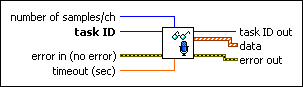number of samples/ch specifies the number of samples per channel to read from the buffer.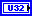task ID is the sound operation from the configured device you want to manipulate or input. You generate task ID with the Sound Input Configure VI.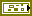error in describes error conditions that occur before this node runs. This input provides standard error in functionality.timeout (sec) specifies the time, in seconds, that the VI waits for the sound operation to complete. This VI returns an error if the time elapses. The default is 10. If you set timeout (sec) to -1, the VI waits indefinitely.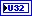task ID out is the manipulated sound operation originally passed to the task ID parameter.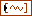data reads any sound data from the internal buffers. For multi-channel sound data, data is an array of waveforms where each element of the array is a single channel.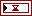t0 is the start time for the first sample read. LabVIEW approximates the initial time that the first sample was read because the sound file does not contain this data.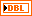dt is the sampling interval. It is the inverse of the sampling rate specified by the Sound Input Config VI.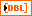Y is the sound data. If the array data type is a floating-point numeric, Y ranges from -1.0 to 1.0. The specified data type determines the range of values for the sound data.error out contains error information. This output provides standard error out functionality.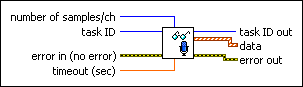number of samples/ch specifies the number of samples per channel to read from the buffer.task ID is the sound operation from the configured device you want to manipulate or input. You generate task ID with the Sound Input Configure VI.error in describes error conditions that occur before this node runs. This input provides standard error in functionality.timeout (sec) specifies the time, in seconds, that the VI waits for the sound operation to complete. This VI returns an error if the time elapses. The default is 10. If you set timeout (sec) to -1, the VI waits indefinitely.task ID out is the manipulated sound operation originally passed to the task ID parameter.data reads any sound data from the internal buffers. For multi-channel sound data, data is an array of waveforms where each element of the array is a single channel.t0 is the start time for the first sample read. LabVIEW approximates the initial time that the first sample was read because the sound file does not contain this data.dt is the sampling interval. It is the inverse of the sampling rate specified by the Sound Input Config VI.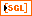Y is the sound data. If the array data type is a floating-point numeric, Y ranges from -1.0 to 1.0. The specified data type determines the range of values for the sound data.error out contains error information. This output provides standard error out functionality.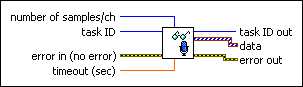number of samples/ch specifies the number of samples per channel to read from the buffer.task ID is the sound operation from the configured device you want to manipulate or input. You generate task ID with the Sound Input Configure VI.error in describes error conditions that occur before this node runs. This input provides standard error in functionality.timeout (sec) specifies the time, in seconds, that the VI waits for the sound operation to complete. This VI returns an error if the time elapses. The default is 10. If you set timeout (sec) to -1, the VI waits indefinitely.task ID out is the manipulated sound operation originally passed to the task ID parameter.data reads any sound data from the internal buffers. For multi-channel sound data, data is an array of waveforms where each element of the array is a single channel.t0 is the start time for the first sample read. LabVIEW approximates the initial time that the first sample was read because the sound file does not contain this data.dt is the sampling interval. It is the inverse of the sampling rate specified by the Sound Input Config VI.Y is the sound data. If the array data type is an 8-bit unsigned integer, Y ranges from 0 to 255. The specified data type determines the range of values for the sound data.error out contains error information. This output provides standard error out functionality.number of samples/ch specifies the number of samples per channel to read from the buffer.task ID is the sound operation from the configured device you want to manipulate or input. You generate task ID with the Sound Input Configure VI.error in describes error conditions that occur before this node runs. This input provides standard error in functionality.timeout (sec) specifies the time, in seconds, that the VI waits for the sound operation to complete. This VI returns an error if the time elapses. The default is 10. If you set timeout (sec) to -1, the VI waits indefinitely.task ID out is the manipulated sound operation originally passed to the task ID parameter.data reads any sound data from the internal buffers. For multi-channel sound data, data is an array of waveforms where each element of the array is a single channel.t0 is the start time for the first sample read. LabVIEW approximates the initial time that the first sample was read because the sound file does not contain this data.dt is the sampling interval. It is the inverse of the sampling rate specified by the Sound Input Config VI.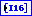Y is the sound data. If the array data type is a 16-bit signed integer, Y ranges from -32768 to 32767. The specified data type determines the range of values for the sound data.error out contains error information. This output provides standard error out functionality.number of samples/ch specifies the number of samples per channel to read from the buffer.task ID is the sound operation from the configured device you want to manipulate or input. You generate task ID with the Sound Input Configure VI.error in describes error conditions that occur before this node runs. This input provides standard error in functionality.timeout (sec) specifies the time, in seconds, that the VI waits for the sound operation to complete. This VI returns an error if the time elapses. The default is 10. If you set timeout (sec) to -1, the VI waits indefinitely.task ID out is the manipulated sound operation originally passed to the task ID parameter.data reads any sound data from the internal buffers. For multi-channel sound data, data is an array of waveforms where each element of the array is a single channel.t0 is the start time for the first sample read. LabVIEW approximates the initial time that the first sample was read because the sound file does not contain this data.dt is the sampling interval. It is the inverse of the sampling rate specified by the Sound Input Config VI.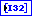Y is the sound data. If the array data type is a 32-bit signed integer, Y ranges from -2147483648 to 2147483647. The specified data type determines the range of values for the sound data.error out contains error information. This output provides standard error out functionality.

## Examples

Refer to the following VIs for examples of using the Sound Input Read VI:

• Continuous Sound Input VI: labview\examples\Graphics and Sound\Sound
• Finite Sound Input VI: labview\examples\Graphics and Sound\Sound
• Simultaneous Sound Input Output VI: labview\examples\Graphics and Sound\Sound